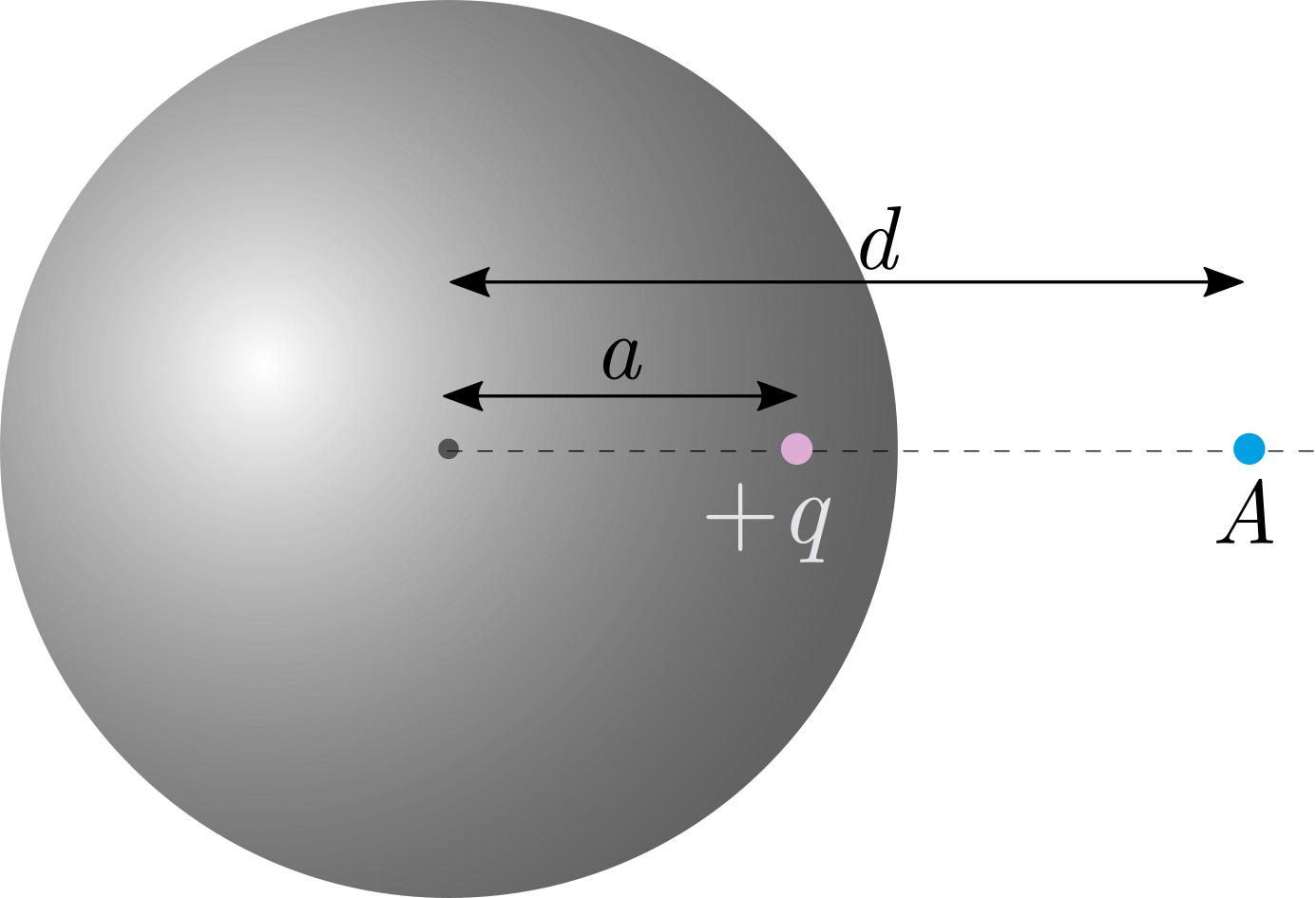# Electric Field Due To Charge And SphereWe have an uncharged, hollow, metal sphere of radius $r$. We place a point charge $+q$ in the sphere at a distance of $a$ from the center.

What is the magnitude of electric field at point $A$ outside the sphere, which is at distance $d$ from the center of the sphere?

×